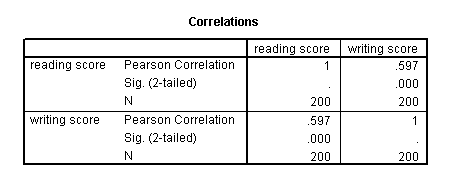## Correlation

A correlation is useful when you want to see the relationship between two (or more) normally distributed interval variables. For example, using the hsb2 data file we can run a correlation between two continuous variables, read and write.

correlations /variables = read write.correlations /variables = female write.In the second example, we will run a correlation between a dichotomous variable, female, and a continuous variable, write. Although it is assumed that the variables are interval and normally distributed, we can include dummy variables when performing correlations.

In the first example above, we see that the correlation between read and write is 0.597. By squaring the correlation and then multiplying by 100, you can determine what percentage of the variability is shared. Let’s round 0.597 to be 0.6, which when squared would be .36, multiplied by 100 would be 36%. Hence read shares about 36% of its variability with write. In the output for the second example, we can see the correlation between write and female is 0.256. Squaring this number yields .065536, meaning that female shares approximately 6.5% of its variability with write.